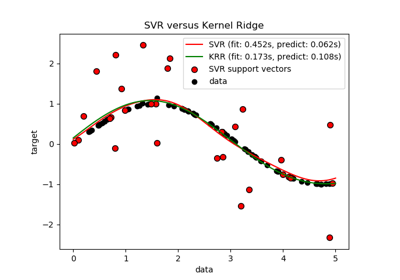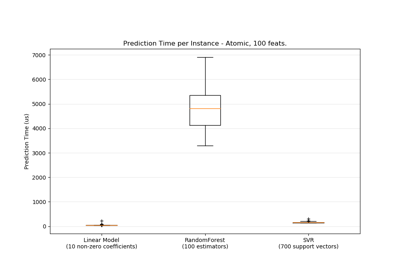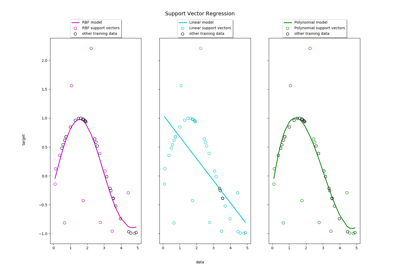# sklearn.svm.SVR¶

class sklearn.svm.SVR(kernel='rbf', degree=3, gamma='auto_deprecated', coef0=0.0, tol=0.001, C=1.0, epsilon=0.1, shrinking=True, cache_size=200, verbose=False, max_iter=-1)[source]

Epsilon-Support Vector Regression.

The free parameters in the model are C and epsilon.

The implementation is based on libsvm.

Read more in the User Guide.

Parameters: kernel : string, optional (default=’rbf’) Specifies the kernel type to be used in the algorithm. It must be one of ‘linear’, ‘poly’, ‘rbf’, ‘sigmoid’, ‘precomputed’ or a callable. If none is given, ‘rbf’ will be used. If a callable is given it is used to precompute the kernel matrix. degree : int, optional (default=3) Degree of the polynomial kernel function (‘poly’). Ignored by all other kernels. gamma : float, optional (default=’auto’) Kernel coefficient for ‘rbf’, ‘poly’ and ‘sigmoid’. Current default is ‘auto’ which uses 1 / n_features, if gamma='scale' is passed then it uses 1 / (n_features * X.var()) as value of gamma. The current default of gamma, ‘auto’, will change to ‘scale’ in version 0.22. ‘auto_deprecated’, a deprecated version of ‘auto’ is used as a default indicating that no explicit value of gamma was passed. coef0 : float, optional (default=0.0) Independent term in kernel function. It is only significant in ‘poly’ and ‘sigmoid’. tol : float, optional (default=1e-3) Tolerance for stopping criterion. C : float, optional (default=1.0) Penalty parameter C of the error term. epsilon : float, optional (default=0.1) Epsilon in the epsilon-SVR model. It specifies the epsilon-tube within which no penalty is associated in the training loss function with points predicted within a distance epsilon from the actual value. shrinking : boolean, optional (default=True) Whether to use the shrinking heuristic. cache_size : float, optional Specify the size of the kernel cache (in MB). verbose : bool, default: False Enable verbose output. Note that this setting takes advantage of a per-process runtime setting in libsvm that, if enabled, may not work properly in a multithreaded context. max_iter : int, optional (default=-1) Hard limit on iterations within solver, or -1 for no limit. support_ : array-like, shape = [n_SV] Indices of support vectors. support_vectors_ : array-like, shape = [nSV, n_features] Support vectors. dual_coef_ : array, shape = [1, n_SV] Coefficients of the support vector in the decision function. coef_ : array, shape = [1, n_features] Weights assigned to the features (coefficients in the primal problem). This is only available in the case of a linear kernel. coef_ is readonly property derived from dual_coef_ and support_vectors_. intercept_ : array, shape =  Constants in decision function.

See also

NuSVR
Support Vector Machine for regression implemented using libsvm using a parameter to control the number of support vectors.
LinearSVR
Scalable Linear Support Vector Machine for regression implemented using liblinear.

Notes

Examples

>>> from sklearn.svm import SVR
>>> import numpy as np
>>> n_samples, n_features = 10, 5
>>> np.random.seed(0)
>>> y = np.random.randn(n_samples)
>>> X = np.random.randn(n_samples, n_features)
>>> clf = SVR(gamma='scale', C=1.0, epsilon=0.2)
>>> clf.fit(X, y)
SVR(C=1.0, cache_size=200, coef0=0.0, degree=3, epsilon=0.2, gamma='scale',
kernel='rbf', max_iter=-1, shrinking=True, tol=0.001, verbose=False)


Methods

 fit(X, y[, sample_weight]) Fit the SVM model according to the given training data. get_params([deep]) Get parameters for this estimator. predict(X) Perform regression on samples in X. score(X, y[, sample_weight]) Returns the coefficient of determination R^2 of the prediction. set_params(**params) Set the parameters of this estimator.
__init__(kernel='rbf', degree=3, gamma='auto_deprecated', coef0=0.0, tol=0.001, C=1.0, epsilon=0.1, shrinking=True, cache_size=200, verbose=False, max_iter=-1)[source]
fit(X, y, sample_weight=None)[source]

Fit the SVM model according to the given training data.

Parameters: X : {array-like, sparse matrix}, shape (n_samples, n_features) Training vectors, where n_samples is the number of samples and n_features is the number of features. For kernel=”precomputed”, the expected shape of X is (n_samples, n_samples). y : array-like, shape (n_samples,) Target values (class labels in classification, real numbers in regression) sample_weight : array-like, shape (n_samples,) Per-sample weights. Rescale C per sample. Higher weights force the classifier to put more emphasis on these points. self : object

Notes

If X and y are not C-ordered and contiguous arrays of np.float64 and X is not a scipy.sparse.csr_matrix, X and/or y may be copied.

If X is a dense array, then the other methods will not support sparse matrices as input.

get_params(deep=True)[source]

Get parameters for this estimator.

Parameters: deep : boolean, optional If True, will return the parameters for this estimator and contained subobjects that are estimators. params : mapping of string to any Parameter names mapped to their values.
predict(X)[source]

Perform regression on samples in X.

For an one-class model, +1 (inlier) or -1 (outlier) is returned.

Parameters: X : {array-like, sparse matrix}, shape (n_samples, n_features) For kernel=”precomputed”, the expected shape of X is (n_samples_test, n_samples_train). y_pred : array, shape (n_samples,)
score(X, y, sample_weight=None)[source]

Returns the coefficient of determination R^2 of the prediction.

The coefficient R^2 is defined as (1 - u/v), where u is the residual sum of squares ((y_true - y_pred) ** 2).sum() and v is the total sum of squares ((y_true - y_true.mean()) ** 2).sum(). The best possible score is 1.0 and it can be negative (because the model can be arbitrarily worse). A constant model that always predicts the expected value of y, disregarding the input features, would get a R^2 score of 0.0.

Parameters: X : array-like, shape = (n_samples, n_features) Test samples. For some estimators this may be a precomputed kernel matrix instead, shape = (n_samples, n_samples_fitted], where n_samples_fitted is the number of samples used in the fitting for the estimator. y : array-like, shape = (n_samples) or (n_samples, n_outputs) True values for X. sample_weight : array-like, shape = [n_samples], optional Sample weights. score : float R^2 of self.predict(X) wrt. y.
set_params(**params)[source]

Set the parameters of this estimator.

The method works on simple estimators as well as on nested objects (such as pipelines). The latter have parameters of the form <component>__<parameter> so that it’s possible to update each component of a nested object.

Returns: self

## Examples using sklearn.svm.SVR¶Comparison of kernel ridge regression and SVRPrediction LatencySupport Vector Regression (SVR) using linear and non-linear kernels